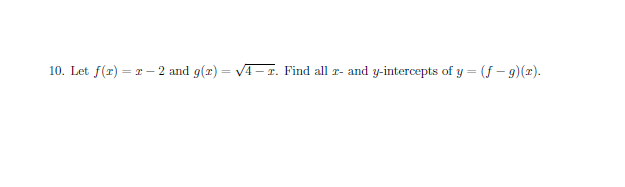# r- 2 and g(x)=- I. Find all r- and y-intercepts of y = (f -g) (x)10. Let f(r)

Questionhelp_outlineImage Transcriptioncloser- 2 and g(x)=- I. Find all r- and y-intercepts of y = (f -g) (x) 10. Let f(r) fullscreen
check_circleExpert Solution
Step 1

First simplify the (f-g)(x) function.

y=x-2-sqrt(4-x)

Step 2

For y intercept plug x=0 and find y.

A...

### Want to see the full answer?

See Solution

#### Want to see this answer and more?

Solutions are written by subject experts who are available 24/7. Questions are typically answered within 1 hour*

See Solution
*Response times may vary by subject and question
Tagged in

### Algebra